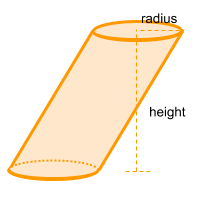Home » Non-polyhedra » Oblique Cylinder

# Volume of an Oblique CylinderVolume =

## Volume calculator for an oblique cylinder

Enter the radius and height of the oblique cylinder to get the volume automatically

## Description, how many faces, edges and vertices are there in an oblique cylinder

The oblique cylinder, is a cylinder where the base and its opposite base are not aligned. It has 3faces just like a regular cylinder, 2 faces that form the base and the opposite base and one face that forms a curved side. 2 curved edges and no vertices.

If the base and the opposite base are aligned, we are in the presence of a regular cylinder.

## Examples of an oblique cylinder

We can find many objects that look like an oblique cylinder, examples of oblique cylinder shaped objects are: the famous Tower of Pisa in Italy or a stack of poker chips which are tilt to one side. Can you think of any other examples? leave a comment in the comment section at the bottom of the page.

## Formula for the volume of an oblique cylinder

To calculate the volume of an oblique cylinder is very simple, we must multiply π (Pi = ~ 3.14) by the radius to the power of two and then multiply by the height. In this case the height forms a 90 degree angle from the opposite base to the position of the base, but outside of it.
You can also use the online calculator to get the oblique cylinder volume automatically.

Volume =